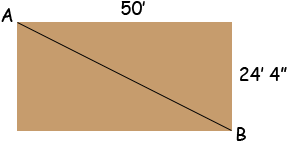SEARCH HOMEMath Central Quandaries & QueriesQuestion from charles: I want to build a garage that is 24 feet 4 inches wide by 50 feet long. can you please tell me what the length of one corner is to the other?Hi Charles,You can use Pythagoras' Theorem to find the distance from A to B. 4 inches is 4/12 = 1/3 = 0.333 feet so

|AB|2 = 502 + 24.3332 = 392.09

and the square root of 392.09 is 55.60 so the distance from A to B is 55.60 feet which is 55 feet 7 1/4 inches.

PennyMath Central is supported by the University of Regina and The Pacific Institute for the Mathematical Sciences.